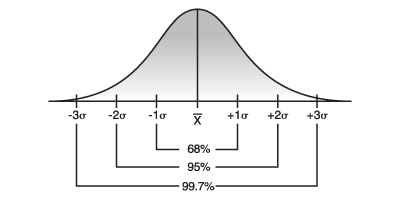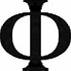Posted on

## Quartiles

Quartile is another term referred to in percentile measure. The total of 100% is partitioned in three places, Q1, Q2, and Q3.

• Lower Quartile is the 25th percentile.  (0.25)
• Median Quartile is the 50th percentile. (0.50)
• Upper Quartile is the 75th percentile.  (0.75)

Example:

Posted on

## Empirical rule – The 68-95-99.7 RuleEmpirical rule

### The 68-95-99.7 RuleFor Normal Distributions

This rule applies generally to a variable X having normal (bell-shaped or mound-shaped) distribution with mean "mu" (the greek letter) and standard deviation "sigma" (the greek letter). However, this rule does not apply to distributions that are not normal.

Posted on

## Hypothesis testing

There are three possible pairs of null and alternative hypotheses

The null hypothesis (denoted) is a statement about the population statistic, such as.

The alternate hypothesis (denoted) is a claim to be tested.

There are three ways to set up a the null and alternate hypothesis.

1. Equal hypothesis verses not equal hypothesis (two-tailed test):= some value:?some value
2. Equal hypothesis verses less than hypothesis (left-tailed test):= some value:some value
3. Equal hypothesis verses greater than hypothesis (right-tailed test):= some value:some value
Posted on

## Pythagoras – three is the perfect numberPythagoras

Pythagoras calls three the perfect number, expressive of “beginning, middle, and end,” wherefore he makes it a symbol of Deity. The world was supposed to be under the rule of three gods, viz. Jupiter (heaven), Neptune (sea), and Pluto (Hades).
Posted on

## Triangles and Simplices

These pointers discuss triangles and their higher-dimensional generalizations (simplices). I am particularly interested in triangulation by which I mean partitioning regions into triangles, tetrahedra, or higher dimensional simplices, for various applications including finite element mesh generation and surface interpolation. (The other meaning of triangulation involves determining locations and distances from certain measurements.) For more material on the first type of triangulation, see the mesh generation section of Geometry in Action or the list of my own triangulation papers. For other kinds of partitions, see the page on dissection

Posted on

## Geometry – 3 basic typesBehavior of lines with a common perpendicular in each of the three types of geometry
• Hyperbolic
• Euclidian
• Ellipitic
Posted on

## Three Types of Triangles

• Scalene triangle: A triangle with no congruent sides
• Isosceles triangle: A triangle with at least two congruent sides
• Equilateral triangle: A triangle with three congruent sides
Posted on

## Golden Ratio – PhiPhi

A straight line is said to have been cut in extreme and mean ratio when, as the whole line is to the greater segment, so is the greater to the less. [Euclid]

The extreme and mean ratio is also known as the golden ratio, or Phi. If the smaller part = 1, and larger part = G, the golden ratio requires that  G is equal approximately 1.6180

### Does the Great Pyramid contain the Golden Ratio?

Assuming that the height of the GP = 146.515 m, and base = 230.363 m, and using simple math we find that half of the base is 115.182 m and the “slant height”  is 186.369 m

Dividing the “slant height” (186.369m) by “half base” (115.182m) gives = 1.6180, which is practically equal to the golden ration!

Posted on

## Sacred Geometry – Flower of Life

By ANDREW MONKMAN [a.monkman@btinternet.com]Flower of Life

The “Flower of Life” can be found in all major religions of the world. It contains the patterns of creation as they emerged from the “Great Void”. Everything is made from the Creator’s thought. After the creation of the Seed of Life the same vortex’s motion was continued, creating the next structure known as the Egg of Life.

Posted on

## Percentage of integers containing digit 3

What percentage of all integers contains at least one instance of the digit three? For example, 13, 31, 33 and 103 all contain the digit "three" at least once. ANSWER!   Answer: How Many Threes? 100% of all integers contain at least one three. What?!? How can this be? The solution is so surprising, it is difficult, if not impossible to believe that 100% of integers contain the digit three at least once.

Posted on

## equivalence relation

 In set theory, a relation that is reflexive, symmetric, & transitive on a set X is called an equivalence relation on X. SImply put as a graph, reflexive me ans there is a loop, symetric means for every directed edge from v to w, there is also a directed edge from w to v, and transitive means for a directed edge x to y, and y to z, there is also an edge from x to z.